# Basins of attraction of certain rational anti-competitive system of difference equations in the plane

## Abstract

We investigate the global asymptotic behavior of solutions of the following anti-competitive system of rational difference equations:

$x n + 1 = γ 1 y n A 1 + x n , y n + 1 = β 2 x n A 2 + B 2 x n + y n ,n=0,1,…,$

where the parameters $γ 1$, $β 2$, $A 1$, $A 2$ and $B 2$ are positive numbers and the initial conditions $( x 0 , y 0 )$ are arbitrary nonnegative numbers. We find the basins of attraction of all attractors of this system, which are the equilibrium points and period-two solutions.

MSC:39A10, 39A11.

## 1 Introduction

A first-order system of difference equations

${ x n + 1 = f ( x n , y n ) , y n + 1 = g ( x n , y n ) , n=0,1,…,( x 0 , y 0 )∈R,$
(1)

where $R⊂ R 2$, $(f,g):R→R,f,g$ are continuous functions is competitive if $f(x,y)$ is non-decreasing in x and non-increasing in y, and $g(x,y)$ is non-increasing in x and non-decreasing in y.

System (1) where the functions f and g have a monotonic character opposite of the monotonic character in competitive system will be called anti-competitive.

We consider the following anti-competitive system of difference equations:

$x n + 1 = γ 1 y n A 1 + x n , y n + 1 = β 2 x n A 2 + B 2 x n + y n ,n=0,1,…,$
(2)

where the parameters $A 1$, $γ 1$, $A 2$, $B 2$ and $β 2$ are positive numbers and the initial conditions $( x 0 , y 0 )$ are arbitrary nonnegative numbers. In the classification of all linear fractional systems in , System (2) was mentioned as System (16, 39).

Competitive and cooperative systems of the form (1) were studied by many authors such as Clark and Kulenović , Clark, Kulenović and Selgrade , Hirsch and Smith , Kulenović and Ladas , Kulenović and Merino , Kulenović and Nurkanović [7, 8], Garić-Demirović, Kulenović and Nurkanović [9, 10], Smith [11, 12] and others.

The study of anti-competitive systems started in  and has advanced since then (see [14, 15]). The principal tool of the study of anti-competitive systems is the fact that the second iterate of the map associated with an anti-competitive system is a competitive map, and so the elaborate theory for such maps developed recently in [4, 16, 17] can be applied.

The main result on the global behavior of System (2) is the following theorem.

Theorem 1 (a) If $β 2 γ 1 ≤ A 1 A 2$, then $E 0 =(0,0)$ is a unique equilibrium, and the basin of attraction of this equilibrium is $B( E 0 )={(x,y):x≥0,y≥0}$ (see Figure 1(a)).

(b) If $β 2 γ 1 − A 1 A 2 >− B 2 [ A 1 2 + γ 1 ( A 2 − A 1 B 2 )]$ and $β 2 γ 1 − A 1 A 2 >0$, then there exist two equilibrium points: $E 0$ which is a repeller and $E +$ which is an interior saddle point, and minimal period-two solutions $A 0 =(0, β 2 γ 1 − A 1 A 2 γ 1 B 2 )$ and $B 0 =( β 2 γ 1 − A 1 A 2 A 1 B 2 ,0)$ which are locally asymptotically stable. There exists a set $C⊂ R = [0,∞)×[0,∞)$ such that $E 0 ∈C$, and $W s ( E + )=C∖ E 0$ is an invariant subset of the basin of attraction of $E +$. The set $C$ is a graph of a strictly increasing continuous function of the first variable on an interval and separates $R$ into two connected and invariant components, namely

$W − := { x ∈ R ∖ C : ∃ x ′ ∈ C with x ⪯ s e x ′ } , W + := { x ∈ R ∖ C : ∃ x ′ ∈ C with x ′ ⪯ s e x } ,$

which satisfy (see Figure 1(b)):

(i) If $( x 0 , y 0 )∈ W +$, then

$lim n → ∞ ( x 2 n , y 2 n )= ( β 2 γ 1 − A 1 A 2 A 1 B 2 , 0 ) = B 0$

and

$lim n → ∞ ( x 2 n + 1 , y 2 n + 1 )= ( 0 , β 2 γ 1 − A 1 A 2 γ 1 B 2 ) = A 0 .$

(ii) If $( x 0 , y 0 )∈ W −$, then

$lim n → ∞ ( x 2 n , y 2 n )= ( 0 , β 2 γ 1 − A 1 A 2 γ 1 B 2 ) = A 0$

and

$lim n → ∞ ( x 2 n + 1 , y 2 n + 1 )= ( β 2 γ 1 − A 1 A 2 A 1 B 2 , 0 ) = B 0 .$

(c) If $0< β 2 γ 1 − A 1 A 2 =− B 2 [ A 1 2 + γ 1 ( A 2 − A 1 B 2 )]$, then (see Figure 1(c))

(i) There exist two equilibrium points: $E 0$ which is a repeller and $E + ∈int(R)$ which is a non-hyperbolic, and an infinite number of minimal period-two solutions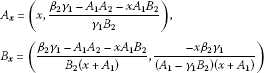for $x∈[0, β 2 γ 1 − A 1 A 2 A 1 B 2 ]$, that belong to the segment of the line (15) in the first quadrant.

(ii) All minimal period-two solutions and the equilibrium $E +$ are stable but not asymptotically stable.

(iii) There exists a family of strictly increasing curves $C +$, $C A x$, $C B x$ for $x∈(0, β 2 γ 1 − A 1 A 2 A 1 B 2 )$ and

$C A 0 = { ( x , y ) : x = 0 , y > 0 } , C B 0 = { ( x , y ) : x > 0 , y = 0 }$

that emanate from $E 0$ and $A x ∈ C A x$, $B x ∈ C B x$ for all $x∈[0, β 2 γ 1 − A 1 A 2 A 1 B 2 )$, such that the curves are pairwise disjoint, the union of all the curves equals $R + 2$. Solutions with initial points in $C +$ converge to $E +$ and solutions with an initial point in $C A x$ have even-indexed terms converging to $A x$ and odd-indexed terms converging to $B x$; solutions with an initial point in $C B x$ have even-indexed terms converging to $B x$ and odd-indexed terms converging to $A x$.

(d) If $0< β 2 γ 1 − A 1 A 2 <− B 2 [ A 1 2 + γ 1 ( A 2 − A 1 B 2 )]$, then System (2) has two equilibrium points: $E 0$ which is a repeller and $E +$ which is locally asymptotically stable, and minimal period-two solutions $A 0$ and $B 0$ which are saddle points. The basin of attraction of the equilibrium point $E +$ is the set

$B( E + )= { ( x , y ) : x > 0 , y > 0 }$

and solutions with an initial point in ${(x,y):x=0,y>0}$ have even-indexed terms converging to $A 0$ and odd-indexed terms converging to $B 0$, solutions with an initial point in ${(x,y):x>0,y=0}$ have even-indexed terms converging to $B 0$ and odd-indexed terms converging to $A 0$ (see Figure 1(d)).

## 2 Preliminaries

We now give some basic notions about systems and maps in the plane of the form (1).

Consider a map $T=(f,g)$ on a set $R⊂ R 2$, and let $E∈R$. The point $E∈R$ is called a fixed point if $T(E)=E$. An isolated fixed point is a fixed point that has a neighborhood with no other fixed points in it. A fixed point $E∈R$ is an attractor if there exists a neighborhood $U$ of E such that $T n (x)→E$ as $n→∞$ for $x∈U$; the basin of attraction is the set of all $x∈R$ such that $T n (x)→E$ as $n→∞$. A fixed point E is a global attractor on a set $K$ if E is an attractor and $K$ is a subset of the basin of attraction of E. If T is differentiable at a fixed point E, and if the Jacobian $J T (E)$ has one eigenvalue with modulus less than one and a second eigenvalue with modulus greater than one, E is said to be a saddle. See  for additional definitions.

Here we give some basic facts about the monotone maps in the plane, see [11, 16, 17, 19]. Now, we write System (2) in the form

$( x y ) n + 1 =T( x y ) n ,$

where the map T is given as

$T:( x y )→( γ 1 y A 1 + x β 2 x A 2 + B 2 x + y )=( f ( x , y ) g ( x , y ) ).$
(3)

The map T may be viewed as a monotone map if we define a partial order on $R 2$ so that the positive cone in this new partial order is the fourth quadrant. Specifically, for $v=( v 1 , v 2 )$, $w=( w 1 , w 2 )∈ R 2$ we say that $v⪯w$ if $v 1 ≤ w 1$ and $w 2 ≤ v 2$. Two points $v,w∈ R + 2$ are said to be related if $v⪯w$ or $w⪯v$. Also, a strict inequality between points may be defined as $v≺w$ if $v⪯w$ and $v≠w$. A stronger inequality may be defined as $v≺≺w$ if $v 1 < w 1$ and $w 2 < v 2$. A map $f:int R + 2 →Int R + 2$ is strongly monotone if $v≺w$ implies that $f(v)≺≺f(w)$ for all $v,w∈Int R + 2$. Clearly, being related is an invariant under iteration of a strongly monotone map. Differentiable strongly monotone maps have Jacobian with constant sign configuration

$[ + − − + ].$

The mean value theorem and the convexity of $R + 2$ may be used to show that T is monotone, as in .

For $x=( x 1 , x 2 )∈ R 2$, define $Q l (x)$ for $l=1,…,4$ to be the usual four quadrants based at x and numbered in a counterclockwise direction, for example, $Q 1 (x)={ y = ( y 1 , y 2 )∈ R 2 : x 1 ≤ y 1 , x 2 ≤ y 2 }$.

The following definition is from .

Definition 1 Let $S$ be a nonempty subset of $R 2$. A competitive map $T:S→S$ is said to satisfy condition (O+) if for every x, y in $S$, $T(x) ⪯ n e T(y)$ implies $x ⪯ n e y$, and T is said to satisfy condition (O−) if for every x, y in $S$, $T(x) ⪯ n e T(y)$ implies $y ⪯ n e x$.

The following theorem was proved by de Mottoni-Schiaffino for the Poincaré map of a periodic competitive Lotka-Volterra system of differential equations. Smith generalized the proof to competitive and cooperative maps .

Theorem 2 Let $S$ be a nonempty subset of $R 2$. If T is a competitive map for which (O+) holds then for all $x∈S$, ${ T n (x)}$ is eventually componentwise monotone. If the orbit of x has compact closure, then it converges to a fixed point of T. If instead (O−) holds, then for all $x∈S$, ${ T 2 n }$ is eventually componentwise monotone. If the orbit of x has compact closure in $S$, then its omega limit set is either a period-two orbit or a fixed point.

The following result is from , with the domain of the map specialized to be the Cartesian product of intervals of real numbers. It gives a sufficient condition for conditions (O+) and (O−).

Theorem 3 Let $R⊂ R 2$ be the Cartesian product of two intervals in $R$. Let $T:R→R$ be a $C 1$ competitive map. If T is injective and $det J T (x)>0$ for all $x∈R$ then T satisfies (O+). If T is injective and $det J T (x)<0$ for all $x∈R$ then T satisfies (O−).

Next two results are from [17, 21].

Theorem 4 Let T be a competitive map on a rectangular region $R⊂ R 2$. Let $x ¯ ∈R$ be a fixed point of T such that $Δ:=R∩int( Q 1 ( x ¯ )∪ Q 3 ( x ¯ ))$ is nonempty (i.e., $x ¯$ is not the NW or SE vertex of $R$), and T is strongly competitive on Δ. Suppose that the following statements are true.

1. a.

The map T has a $C 1$ extension to a neighborhood of $x ¯$.

2. b.

The Jacobian matrix of T at $x ¯$ has real eigenvalues λ, μ such that $0<|λ|<μ$, where $|λ|<1$, and the eigenspace $E λ$ associated with λ is not a coordinate axis.

Then there exists a curve $C⊂R$ through $x ¯$ that is invariant and a subset of the basin of attraction of $x ¯$, such that $C$ is tangential to the eigenspace $E λ$ at $x ¯$, and $C$ is the graph of a strictly increasing continuous function of the first coordinate on an interval. Any endpoints of $C$ in the interior of $R$ are either fixed points or minimal period-two points. In the latter case, the set of endpoints of $C$ is a minimal period-two orbit of T.

Theorem 5 (Kulenović & Merino)

Let $I 1$, $I 2$ be intervals in $R$ with endpoints $a 1$, $a 2$ and $b 1$, $b 2$ with endpoints respectively, with $a 1 < a 2$ and $b 1 < b 2$, where $−∞≤ a 1 < a 2 ≤∞$ and $−∞≤ b 1 < b 2 ≤∞$. Let T be a competitive map on a rectangle $R = I 1 × I 2$ and $x ¯ ∈int(R)$. Suppose that the following hypotheses are satisfied:

1. 1.

$T(int(R))⊂int(R)$ and T is strongly competitive on $int(R)$.

2. 2.

The point $x ¯$ is the only fixed point of T in $( Q 1 ( x ¯ )∪ Q 3 ( x ¯ ))∩int(R)$.

3. 3.

The map T is continuously differentiable in a neighborhood of $x ¯$.

4. 4.

At least one of the following statements is true:

5. a.

T has no minimal period two orbits in $( Q 1 ( x ¯ )∪ Q 3 ( x ¯ ))∩int(R)$.

6. b.

$det J T ( x ¯ )>0$ and $T(x)= x ¯$ only for $x= x ¯$.

7. 5.

$x ¯$ is a saddle point.

Then the following statements are true.

1. (i)

The stable manifold $W s ( x ¯ )$ is connected and it is the graph of a continuous increasing curve with endpoints in $∂R$. $int(R)$ is divided by the closure of $W s ( x ¯ )$ into two invariant connected regions $W +$ (“below the stable set”), and $W −$ (“above the stable set”), where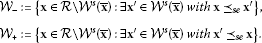2. (ii)

The unstable manifold $W u ( x ¯ )$ is connected, and it is the graph of a continuous decreasing curve.

3. (iii)

For every $x∈ W +$, $T n (x)$ eventually enters the interior of the invariant set $Q 4 ( x ¯ )∩R$, and for every $x∈ W −$, $T n (x)$ eventually enters the interior of the invariant set $Q 2 ( x ¯ )∩R$.

4. (iv)

Let $m∈ Q 2 ( x ¯ )$ and $M∈ Q 4 ( x ¯ )$ be the endpoints of $W u ( x ¯ )$, where $m ⪯ s e x ¯ ⪯ s e M$. For every $x∈ W −$ and every $z∈R$ such that $m ⪯ s e z$, there exists $m∈N$ such that $T m (x) ⪯ s e z$, and for every $x∈ W +$ and every $z∈R$ such that $z ⪯ s e M$, there exists $m∈N$ such that $M ⪯ s e T m (x)$.

## 3 Linearized stability analysis

Lemma 1

1. (i)

If $β 2 γ 1 − A 1 A 2 ≤0$, then System (2) has a unique equilibrium point $E 0 =(0,0)$.

2. (ii)

If $β 2 γ 1 − A 1 A 2 >0$, then System (2) has two equilibrium points $E 0$ and $E + =( x ¯ , y ¯ )$, $x ¯ >0$, $y ¯ >0$.

Proof The equilibrium point $E( x ¯ , y ¯ )$ of System (2) satisfies the following system of equations:

$x ¯ = γ 1 y ¯ A 1 + x ¯ , y ¯ = β 2 x ¯ A 2 + B 2 x ¯ + y ¯ .$
(4)

It is easy to see that $E 0 =(0,0)$ is one equilibrium point for all values of the parameters, and $E + =( x ¯ , y ¯ )$ is a positive equilibrium point if $β 2 γ 1 − A 1 A 2 >0$. Indeed, substituting $y ¯$ from the first equation in (4) in the second equation in (4), we obtain that $x ¯$ satisfies the following equation:

$f(x)= x 3 +(2 A 1 + B 2 γ 1 ) x 2 + ( A 1 2 + A 1 B 2 γ 1 + A 2 γ 1 ) x+ γ 1 ( A 1 A 2 − β 2 γ 1 )=0.$
(5)

By using Descartes’ theorem, we have that equation (5) has one positive equilibrium if the condition

$β 2 γ 1 − A 1 A 2 >0$
(6)

is satisfied, i.e., $β 2 γ 1 > A 1 A 2$. □

Theorem 6

1. (i)

If $β 2 γ 1 < A 1 A 2$, then $E 0$ is locally asymptotically stable.

2. (ii)

If $β 2 γ 1 = A 1 A 2$, then $E 0$ is non-hyperbolic.

3. (iii)

If $β 2 γ 1 > A 1 A 2$, then $E 0$ is a repeller.

Proof The map T associated to System (2) is of the form (3). The Jacobian matrix of T at the equilibrium $E=( x ¯ , y ¯ )$ is

$J T ( x ¯ , y ¯ )=( − γ 1 y ¯ ( A 1 + x ¯ ) 2 γ 1 A 1 + x ¯ β 2 ( A 2 + y ¯ ) ( A 2 + B 2 x ¯ + y ¯ ) 2 − β 2 x ¯ ( A 2 + B 2 x ¯ + y ¯ ) 2 )$
(7)

and

$J T (0,0)=( 0 γ 1 A 1 β 2 A 2 0 ).$

The corresponding characteristic equation has the following form:

$λ 2 − β 2 γ 1 A 1 A 2 =0,$

from which $λ 1 , 2 =± β 2 γ 1 A 1 A 2$.

1. (i)

If $β 2 γ 1 < A 1 A 2$, then $| λ 1 , 2 |<1$, i.e., $E 0$ is locally asymptotically stable.

2. (ii)

If $β 2 γ 1 = A 1 A 2$, then $| λ 1 , 2 |=1$, which implies that $E 0$ is non-hyperbolic.

3. (iii)

If $β 2 γ 1 > A 1 A 2$, then $| λ 1 , 2 |>1$, which implies that $E 0$ is a repeller.

□

Theorem 7

1. (1)

Assume that $β 2 γ 1 > A 1 A 2$ and

$β 2 γ 1 − A 1 A 2 >− B 2 [ A 1 2 + γ 1 ( A 2 − A 1 B 2 ) ] .$
(8)

Then the positive equilibrium $E +$ is a saddle point.

1. (2)

Assume that

$0< β 2 γ 1 − A 1 A 2 =− B 2 [ A 1 2 + γ 1 ( A 2 − A 1 B 2 ) ] .$
(9)

Then the positive equilibrium $E +$ is a non-hyperbolic point and

$x ¯ =− A 1 + γ 1 ( A 1 B 2 − A 2 ) , y ¯ = ( − A 1 + γ 1 ( A 1 B 2 − A 2 ) ) γ 1 ( A 1 B 2 − A 2 ) γ 1 .$
1. (3)

Assume that

$0< β 2 γ 1 − A 1 A 2 <− B 2 [ A 1 2 + γ 1 ( A 2 − A 1 B 2 ) ] .$
(10)

Then the positive equilibrium $E +$ is locally asymptotically stable.

Proof The Jacobian matrix of T at the equilibrium $E + =( x ¯ , y ¯ )$ is of the form (7) and the corresponding characteristic equation has the following form:

$λ 2 −pλ+q=0,$

where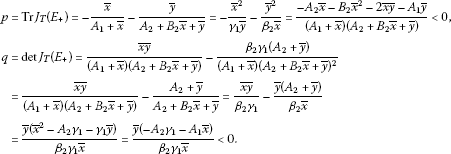Hence, for $E + =( x ¯ , y ¯ )$, we have $p<0$, $q<0$, so $p 2 −4q>0$. Since

$p − q − 1 = − x ¯ 2 γ 1 y ¯ − y ¯ 2 β 2 x ¯ − x ¯ y ¯ β 2 γ 1 + y ¯ ( A 2 + y ¯ ) β 2 x ¯ − 1 = ( 4 ) − x ¯ 2 γ 1 y ¯ − y ¯ 2 β 2 x ¯ − x ¯ y ¯ β 2 γ 1 + ( 1 − B 2 y ¯ β 2 ) − 1 = − x ¯ 2 γ 1 y ¯ − y ¯ 2 β 2 x ¯ − x ¯ y ¯ β 2 γ 1 − B 2 y ¯ β 2 < 0 ,$

we obtain

$|p|{ > | 1 + q | , = | 1 + q | , < | 1 + q | ⇔1+p+q{ < 0 , = 0 , > 0 .$

Similarly,

$1 + p + q = 1 − x ¯ A 1 + x ¯ − y ¯ A 2 + B 2 x ¯ + y ¯ + x ¯ y ¯ ( A 1 + x ¯ ) ( A 2 + B 2 x ¯ + y ¯ ) − A 2 + y ¯ A 2 + B 2 x ¯ + y ¯ = − A 2 x ¯ + y ¯ ( A 1 + x ¯ ) − A 1 B 2 x ¯ ( x ¯ + A 1 ) ( A 2 + B 2 x ¯ + y ¯ ) = ( 4 ) − x ¯ γ 1 ( x ¯ + A 1 ) ( A 2 + B 2 x ¯ + y ¯ ) ϕ ( x ¯ ) ,$

where

Now, for the positive equilibrium, it holdsIf $A 1 2 + γ 1 ( A 2 − A 1 B 2 )≥0$, then $ϕ(x)>0$ for all $x>0$, which implies that $E +$ is a saddle point. If $A 1 2 + γ 1 ( A 2 − A 1 B 2 )<0$, then $ϕ(x)=0$ for $x ± =− A 1 ± γ 1 ( A 1 B 2 − A 2 )$ ($x − <0$, $x + >0$).

Now we have three cases: $x + < x ¯$, $x + = x ¯$ or $x ¯ < x +$. Functions $f(x)$ and $ϕ(x)$ are increasing for $x>0$.

1. (1)

If $x + < x ¯$, then $0=ϕ( x + )<ϕ( x ¯ )$, i.e., $1+p+q<0$ and $f( x + ). So,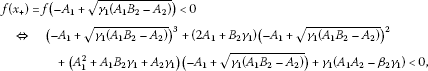from which it follows

$γ 1 B 2 ( A 1 B 2 − A 2 )<( β 2 γ 1 − A 1 A 2 )+ A 1 2 B 2 ,$

i.e.,

$β 2 γ 1 >( A 1 − γ 1 B 2 )( A 2 − A 1 B 2 ).$
(11)

Now we have

$β 2 γ 1 − A 1 A 2 >− B 2 [ A 1 2 + γ 1 ( A 2 − A 1 B 2 ) ] ,$

so we can see that the conditions (8) and (6) are sufficient for $E + =( x ¯ , y ¯ )$ to be a saddle point.

1. (2)

If $x + = x ¯$, then $0=ϕ( x + )=ϕ( x ¯ )$, hence $1+p+q=0$, i.e.,

$f( x + )=f( x ¯ )=f ( − A 1 + γ 1 ( A 1 B 2 − A 2 ) ) =0,$

from which

$β 2 γ 1 =( A 1 − γ 1 B 2 )( A 2 − A 1 B 2 ).$
(12)

If conditions (12) and (6) are satisfied, then

$β 2 γ 2 − A 1 A 2 =− B 2 [ A 1 2 + γ 1 ( A 2 − A 1 B 2 ) ] >0$

holds, i.e., $E + =( x ¯ , y ¯ )$ is a non-hyperbolic point of the form

$x ¯ = x + =− A 1 + γ 1 ( A 1 B 2 − A 2 ) , y ¯ = ( − A 1 + γ 1 ( A 1 B 2 − A 2 ) ) γ 1 ( A 1 B 2 − A 2 ) γ 1 .$
1. (3)

If $x ¯ < x +$, then $ϕ( x ¯ )<ϕ( x + )=0$ and

$0=f( x ¯ )

from which

$β 2 γ 1 <( A 1 − γ 1 B 2 )( A 2 − A 1 B 2 ).$
(13)

Hence, if conditions (13) and (6) are satisfied, then

$0< β 2 γ 2 − A 1 A 2 <− B 2 [ A 1 2 + γ 1 ( A 2 − A 1 B 2 ) ]$

holds, so $E +$ is a locally asymptotically stable. □

## 4 Periodic character of solutions

In this section, we give the existence and local stability of period-two solutions.

Lemma 2 Assume that $β 2 γ 1 > A 1 A 2$. Then System (2) has the following minimal period-two solutions:

$A 0 = ( 0 , β 2 γ 1 − A 1 A 2 γ 1 B 2 ) and B 0 = ( β 2 γ 1 − A 1 A 2 A 1 B 2 , 0 ) .$
(14)

If

$0< β 2 γ 1 − A 1 A 2 =− B 2 [ A 1 2 + γ 1 ( A 2 − A 1 B 2 ) ] ,$

then System (2) has an infinite number of minimal period-two solutions of the formfor $x∈[0, β 2 γ 1 − A 1 A 2 A 1 B 2 ]$, located along the line

$H = { ( x , y ) : x A 1 + γ 1 y + A 1 2 + γ 1 ( A 2 − A 1 B 2 ) = 0 , x ∈ [ 0 , β 2 γ 1 − A 1 A 2 A 1 B 2 ] } .$
(15)

Proof The second iterate of T is (25). Equilibrium curves of the map $T 2 (x,y)$ are

$C 1 T 2 = { ( x , y ) ∈ [ 0 , ∞ ) 2 : x β 2 γ 1 ( x + A 1 ) = x ( y + A 2 + x B 2 ) ( A 1 2 + x A 1 + y γ 1 ) }$
(16)

and

$C 2 T 2 = { ( x , y ) ∈ [ 0 , ∞ ) 2 : y β 2 γ 1 ( y + A 2 + x B 2 ) = y ( A 1 A 2 2 + x 2 β 2 + x A 2 2 + x 2 A 2 B 2 + x y A 2 + x β 2 A 1 + y A 1 A 2 + y 2 γ 1 B 2 + y γ 1 A 2 B 2 + x A 1 A 2 B 2 + x y γ 1 B 2 2 ) } .$
(17)

We get period-two solutions as the intersection point of equilibrium curves (16) and (17) in the first quadrant. If $x≠0$, $y=0$, then System (16), (17) is reduced to the equation

$β 2 γ 1 (x+ A 1 )= A 1 ( A 2 +x B 2 )(x+ A 1 ),$

and the positive solution of this equation is

If $x=0$, $y≠0$, then System (16), (17) is reduced to the equation

$β 2 γ 1 (y+ A 2 )=(y+ A 2 )( A 1 A 2 +y γ 1 B 2 ),$

with the positive solution

On the other hand, if $x>0$, $y>0$, then we have

$β 2 γ 1 ( x + A 1 ) = ( y + A 2 + x B 2 ) ( A 1 2 + x A 1 + y γ 1 ) β 2 γ 1 ( y + A 2 + x B 2 ) = A 1 A 2 2 + x 2 β 2 + x A 2 2 + x 2 A 2 B 2 + x y A 2 + x β 2 A 1 + y A 1 A 2 β 2 γ 1 ( y + A 2 + x B 2 ) = + y 2 γ 1 B 2 + y γ 1 A 2 B 2 + x A 1 A 2 B 2 + x y γ 1 B 2 2 } ,$

that is

$(x+ A 1 )( β 2 γ 1 − A 1 A 2 )=(y+x B 2 ) ( A 1 2 + x A 1 + y γ 1 ) +y γ 1 A 2$
(18)

and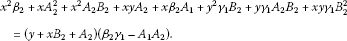(19)

Therefore, it must be $( β 2 γ 1 − A 1 A 2 )>0$ in order to get any positive solution. By eliminating the term $( β 2 γ 1 − A 1 A 2 )$ from (18) and using condition (9), we get

$(y+x B 2 + A 1 B 2 ) ( y γ 1 + x A 1 + A 1 2 + γ 1 A 2 − γ 1 A 1 B 2 ) =0,$

which implies

$y γ 1 +x A 1 + A 1 2 + γ 1 ( A 2 − A 1 B 2 )=0,$

hence

$y=− 1 γ 1 ( x A 1 + A 1 2 + γ 1 ( A 2 − A 1 B 2 ) ) , γ 1 ≠0.$
(20)

Now, by eliminating y and the term $( A 1 A 2 − β 2 γ 1 )$ from (19), we get the identity

$(x+ A 1 )(x+ A 1 − γ 1 B 2 ) β 2 γ 1 − ( A 2 − A 1 B 2 ) ( A 1 − γ 1 B 2 ) γ 1 =0.$

If $x= γ 1 B 2 − A 1$, we have

$y=− 1 γ 1 ( x A 1 + A 1 2 + γ 1 ( A 2 − A 1 B 2 ) ) =− A 2 <0, γ 1 ≠0.$

So, periodic solutions are located along line (15) with endpoints given by (14) using conditions (9). It is easy to see that $A x , B x ∈H$ if $β 2 γ 1 − A 1 A 2 =− B 2 [ A 1 2 + γ 1 ( A 2 − A 1 B 2 )]$. □

Let $(x,y)∈H$, then the corresponding Jacobian matrix of the map $T 2$ has the following form:

$J T 2 H (x,y)=( a b c d ),$
(21)

where $a:= F x (x,y)$, $b:= F y (x,y)$, $c:= G x (x,y)$, $d:= G y (x,y)$.

Lemma 3 Assume that $0< β 2 γ 1 − A 1 A 2 =− B 2 [ A 1 2 + γ 1 ( A 2 − A 1 B 2 )]$. Then the following statements are true.

1. (a)

The points $A x , B x ∈H$ are non-hyperbolic fixed points for the map $T 2$, and both of them have eigenvalues $λ 1 =1$ and $λ 2 ∈(0,1)$.

2. (b)

Eigenvectors corresponding to the eigenvalues $λ 1$ and $λ 2$ are not parallel to coordinate axes.

Proof (a) From (15) we have $y H ′ (x)=− A 1 γ 1 <0$. Since

$H= { ( x , y ) ∈ [ 0 , ∞ ) 2 : F ( x , y ) = x } = { ( x , y ) ∈ [ 0 , ∞ ) 2 : G ( x , y ) = y } ,$

by implicit differentiation of equations $F(x,y)=x$ and $G(x,y)=y$ at the point $(x,y)∈H$, we obtain

$y H ′ (x)= 1 − a b = c 1 − d =− A 1 γ 1 <0.$
(22)

Since $a>0$, $b<0$, $c<0$ and $d>0$, from (22), we get

$0
(23)

The characteristic polynomial of the matrix (21) at the point $(x,y)∈H$ is of the form

$P(λ)= λ 2 −(a+d)λ+(ad−bc).$

Now, using (22) we have $(1−a)(1−d)=bc$, and since

$P(1)=1−(a+d)+(ad−bc)=0,$

we get $λ 1 =1$, and due to Vieta’s formulas and condition (23), it follows

$0< λ 1 + λ 2 =1+ λ 2 =a+d<2,$

i.e., $0< λ 2 <1$.

(b) Eigenvectors corresponding to the eigenvalues $λ 1$ and $λ 2$ are $v 1 =(1−d,c)$ and $v 2 =(a−1,c)$. By condition (23) it is easy to see that these vectors are not parallel to the coordinate axes. □

Lemma 4 The periodic points $A 0$ and $B 0$ given by (14) are

1. (a)

locally asymptotically stable if $β 2 γ 1 − A 1 A 2 >− B 2 [ A 1 2 + γ 1 ( A 2 − A 1 B 2 )]$ and $β 2 γ 1 > A 1 A 2$,

2. (b)

non-hyperbolic if $0< β 2 γ 1 − A 1 A 2 =− B 2 [ A 1 2 + γ 1 ( A 2 − A 1 B 2 )]$,

3. (c)

saddle points if $0< β 2 γ 1 − A 1 A 2 <− B 2 [ A 1 2 + γ 1 ( A 2 − A 1 B 2 )]$.

Proof We have that

$J T 2 ( β 2 γ 1 − A 1 A 2 A 1 B 2 , 0 ) =( A 1 A 2 β 2 γ 1 ( β 2 γ 1 − A 1 A 2 ) ( A 1 2 A 2 − A 1 3 B 2 − β 2 γ 1 2 B 2 − β 2 γ 1 A 1 ) β 2 γ 1 A 1 B 2 ( A 1 2 B 2 + β 2 γ 1 − A 1 A 2 ) 0 β 2 γ 1 2 A 1 B 2 2 ( A 1 2 B 2 + β 2 γ 1 − A 1 A 2 ) ( β 2 γ 1 − A 1 A 2 + γ 1 A 2 B 2 ) )$

and characteristic eigenvalues are

$λ 1 = A 1 A 2 β 2 γ 1 <1and λ 2 = β 2 γ 1 2 A 1 B 2 2 ( β 2 γ 1 − A 1 A 2 + γ 1 A 2 B 2 ) ( B 2 A 1 2 − A 2 A 1 + β 2 γ 1 ) .$

Now,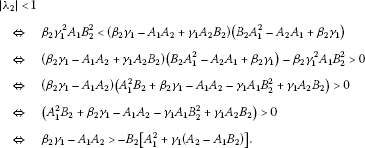Therefore,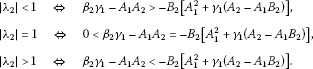On the other hand, we have

$J T 2 ( 0 , β 2 γ 1 − A 1 A 2 γ 1 B 2 ) =( β 2 γ 1 2 A 1 B 2 2 ( A 1 2 B 2 + β 2 γ 1 − A 1 A 2 ) ( β 2 γ 1 − A 1 A 2 + γ 1 A 2 B 2 ) 0 ( β 2 γ 1 − A 1 A 2 ) ( A 1 A 2 2 − γ 1 A 2 2 B 2 − β 2 γ 1 A 2 − β 2 γ 1 A 1 B 2 ) β 2 γ 1 2 B 2 ( β 2 γ 1 − A 1 A 2 + γ 1 A 2 B 2 ) A 1 A 2 β 2 γ 1 )$

and the corresponding eigenvalues are

$λ 1 = A 1 A 2 β 2 γ 1 <1and λ 2 = β 2 γ 1 2 A 1 B 2 2 ( β 2 γ 1 − A 1 A 2 + γ 1 A 2 B 2 ) ( B 2 A 1 2 − A 2 A 1 + β 2 γ 1 ) ,$

so it comes to the same conclusion! □

## 5 Global results

In this section, we present the results on the global dynamics of System (2).

Lemma 5 Every solution of System (2) satisfies

1. 1.

$x n ≤ γ 1 A 1 ⋅ β 2 B 2$, $y n ≤ β 2 B 2$, $n=2,3,…$.

2. 2.

If $β 2 γ 1 < A 1 A 2$, then $lim n → ∞ x n =0$, $lim n → ∞ y n =0$.

The map T satisfies:

1. 3.

$T(B)⊆B$, where $B=[0, γ 1 A 1 ⋅ β 2 B 2 ]×[0, β 2 B 2 ]$, that is, $B$ is an invariant box.

2. 4.

$T(B)$ is an attracting box, that is $T ( [ 0 , ∞ ) 2 )⊆B$.

Proof From System (2), we have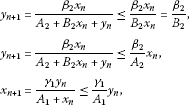for $n=0,1,2,…$ , and

$x n + 1 ≤ γ 1 A 1 y n ≤ γ 1 A 1 ⋅ β 2 B 2$

for $n=1,2,…$ . Furthermore, we get

$x n ≤ γ 1 A 1 y n − 1 ≤ γ 1 β 2 A 1 A 2 x n − 2 ,$

i.e.,

$x 2 n ≤ ( γ 1 β 2 A 1 A 2 ) n x 0 , x 2 n + 1 ≤ ( γ 1 β 2 A 1 A 2 ) n x 1 ,$

so it follows that $lim n → ∞ x n =0$, $lim n → ∞ y n =0$ if $β 2 γ 1 < A 1 A 2$.

Proof of 3. and 4. is an immediate checking. □

Lemma 6 The map $T 2$ is injective and $det J T 2 (x,y)>0$, for all $x≥0$ and $y≥0$.

Proof (i) Here we prove that map T is injective, which implies that $T 2$ is injective. Indeed, $T ( x 1 y 1 ) =T ( x 2 y 2 )$ implies that

$A 1 ( y 1 − y 2 )= x 1 y 2 − x 2 y 1 , A 2 ( x 1 − x 2 )= x 2 y 1 − x 1 y 2 .$
(24)

By solving System (24) with respect to $x 1$, $x 2$ or $y 1$, $y 2$, we obtain that $( x 1 , y 1 )=( x 2 , y 2 )$.

1. (ii)

The map $T 2 (x,y)= ( F ( x , y ) G ( x , y ) )$ is of the form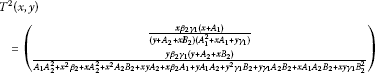(25)

and

$J T 2 (x,y)=( F x F y G x G y ),$

where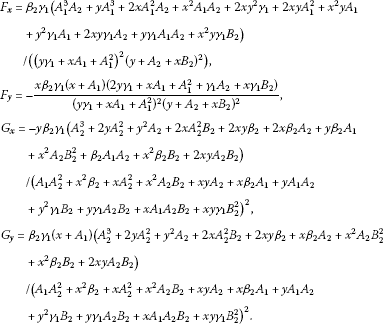Now, we obtain

$det J T 2 (x,y)= F x G y − F y G x =UV,$

where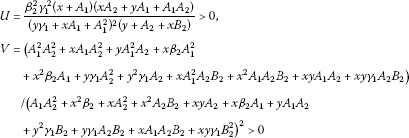and the Jacobian matrix of $T 2 (x,y)$ is invertible for all $x≥0$ and $y≥0$. □

Corollary 1 The competitive map $T 2$ satisfies the condition (O+). Consequently, the sequences ${ x 2 n }$, ${ x 2 n + 1 }$, ${ y 2 n }$, ${ y 2 n + 1 }$ of every solution of System (2) are eventually monotone.

Proof It immediately follows from Lemma 6, Theorem 2 and 3. □

Lemma 7 Assume $β 2 γ 1 − A 1 A 2 >0$. System (2) has period-two solutions (14) and

1. (a)

If $( x 0 , y 0 )=(x,0)$, $x>0$, then

$lim n → ∞ T 2 n (x,0)= ( β 2 γ 1 − A 1 A 2 A 1 B 2 , 0 ) = B 0$

and

$lim n → ∞ T 2 n + 1 (x,0)= ( 0 , β 2 γ 1 − A 1 A 2 γ 1 B 2 ) = A 0 .$
1. (b)

If $( x 0 , y 0 )=(0,y)$, $y>0$, then

$lim n → ∞ T 2 n (0,y)= ( 0 , β 2 γ 1 − A 1 A 2 γ 1 B 2 ) = A 0$

and

$lim n → ∞ T 2 n + 1 (x,0)= ( β 2 γ 1 − A 1 A 2 A 1 B 2 , 0 ) = B 0 .$

Proof (a) For all $x>0$, $x≠ β 2 γ 1 − A 1 A 2 A 1 B 2$, we have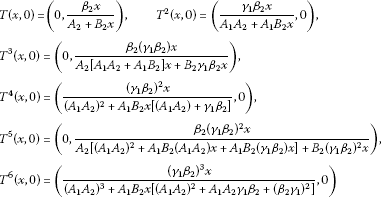and by induction,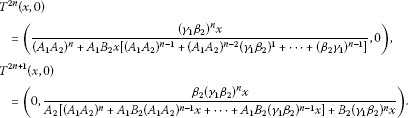Now, we have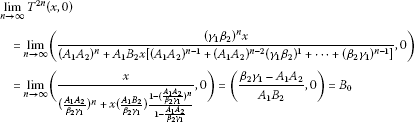and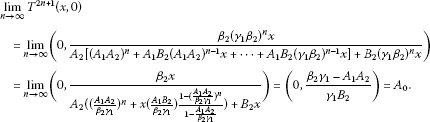□

Lemma 8 The map $T 2$ associated to System (2) satisfies the following:

$T 2 (x,y)=( x ¯ , y ¯ )only for(x,y)=( x ¯ , y ¯ ).$

Proof Since $T 2$ is injective, then $T 2 (x,y)=( x ¯ , y ¯ )= T 2 ( x ¯ , y ¯ )⇒(x,y)=( x ¯ , y ¯ )$. □

Proof of Theorem 1 Case 1 $β 2 γ 1 ≤ A 1 A 2$

Equilibrium $E 0$ is unique (see Lemma 1), and by Lemma 5, every solution of System (2) belongs to

$B=[ 0 , β 2 γ 1 A 1 B 2 ]×[ 0 , β 2 B 2 ],$

which is an invariant box. In view of Corollary 1 and Theorem 2, every solution converges to minimal period-two solutions or $E 0$. System (2) has no minimal period-two solutions (Lemma 2). So, every solution of System (2) converges to $E 0$.

Case 2 $β 2 γ 1 − A 1 A 2 >− B 2 [ A 1 2 + γ 1 ( A 2 − A 1 B 2 )]$ and $β 2 γ 1 − A 1 A 2 >0$

By Lemmas 1, 2, 4 and Theorems 6 and 7, there exist two equilibrium points: $E 0$ which is a repeller and $E +$ which is a saddle point, and minimal period-two solutions $A 0$ and $B 0$ which are locally asymptotically stable. Clearly $T 2$ is strongly competitive and it is easy to check that the points $A 0$ and $B 0$ are locally asymptotically stable for $T 2$ as well. System (2) can be decomposed into the system of the even-indexed and odd-indexed terms as follows:

${ x 2 n + 1 = γ 1 y 2 n A 1 + x 2 n , x 2 n = γ 1 y 2 n − 1 A 1 + x 2 n − 1 , y 2 n + 1 = β 2 x 2 n A 2 + B 2 x 2 n + y 2 n , y 2 n = β 2 x 2 n − 1 A 2 + B 2 x 2 n − 1 + y 2 n − 1 , n = 1 , 2 , … .$

The existence of the set $C$ with the stated properties follows from Lemmas 6, 2, 7, 8, Corollary 1, Theorems 4 and 5.

Case 3 $0< β 2 γ 1 − A 1 A 2 =− B 2 [ A 1 2 + γ 1 ( A 2 − A 1 B 2 )]$

Cases (i) and (ii) from (c) in Theorem 1 are the consequence of Lemmas 1, 2, 4 and Theorems 6 and 7.

Since $T 2$ is strongly competitive and points $A x$ and $B x$, for all $x∈[0, β 2 γ 1 − A 1 A 2 A 1 B 2 )$, are non-hyperbolic points of the map $T 2$, by Lemmas 1, 6, 2, 3, 7, Corollary 1, Theorems 2, 5, 6 and 7, it follows that all conditions of Theorem 4 are satisfied for the map $T 2$ with $R = [0,∞)×[0,∞)$. By Lemma 7, it is clear that

$C A 0 = { ( x , y ) : x = 0 , y > 0 } and C B 0 = { ( x , y ) : x > 0 , y = 0 } .$

Case 4 $0< β 2 γ 1 − A 1 A 2 <− B 2 [ A 1 2 + γ 1 ( A 2 − A 1 B 2 )]$

Lemma 2 implies that System (2) has minimal period-two solutions (14). Furthermore, Corollary 1 and Theorem 2 imply that all solutions of System (2) converge to an equilibrium or minimal period-two solutions, and since, by Theorem 6, $E 0$ is a repeller, all solutions converge to $E +$ (which is, in view of Theorem 7, locally asymptotically stable) or minimal period-two solutions (14). The points $A 0$ and $B 0$ are saddle points of the strongly competitive map $T 2$; and by Lemma 7, the stable manifold of $A 0$ (under $T 2$) is

$B( A 0 )= { ( x , y ) : x = 0 , y > 0 }$

and the stable manifold of $B 0$ (under $T 2$) is

$B( B 0 )= { ( x , y ) : x > 0 , y = 0 }$

and each of these stable manifolds is unique. This implies that the basin of attraction of the equilibrium point $E +$ is the set

$B( E + )= { ( x , y ) : x > 0 , y > 0 } ,$

and Lemma 7 completes the conclusion (d) of Theorem 1. □

## References

1. 1.

Camouzis E, Kulenović MRS, Ladas G, Merino O: Rational systems in the plane - open problems and conjectures. J. Differ. Equ. Appl. 2009, 15: 303–323. 10.1080/10236190802125264

2. 2.

Clark D, Kulenović MRS: On a coupled system of rational difference equations. Comput. Math. Appl. 2002, 43: 849–867. 10.1016/S0898-1221(01)00326-1

3. 3.

Clark CA, Kulenović MRS, Selgrade JF: On a system of rational difference equations. J. Differ. Equ. Appl. 2005, 11: 565–580. 10.1080/10236190412331334464

4. 4.

Hirsch M, Smith H: Monotone Dynamical Systems. Ordinary Differential Equations 2. In Handbook of Differential Equations. Elsevier, Amsterdam; 2005:239–357.

5. 5.

Kulenović MRS, Ladas G: Dynamics of Second Order Rational Difference Equations with Open Problems and Conjectures. Chapman and Hall/CRC, Boca Raton; 2001.

6. 6.

Kulenović MRS, Merino O: Discrete Dynamical Systems and Difference Equations with Mathematica. Chapman and Hall/CRC, Boca Raton; 2002.

7. 7.

Kulenović MRS, Nurkanović M: Asymptotic behavior of a system of linear fractional difference equations. J. Inequal. Appl. 2005, 2005: 127–144.

8. 8.

Kulenović MRS, Nurkanović M: Asymptotic behavior of a competitive system of linear fractional difference equations. Adv. Differ. Equ. 2006, 2006: 1–13.

9. 9.

Brett A, Garić-Demirović M, Kulenović MRS, Nurkanović M: Global behavior of two competitive rational systems of difference equations in the plane. Commun. Appl. Nonlinear Anal. 2009, 16: 1–18.

10. 10.

Garic-Demirović M, Kulenović MRS, Nurkanović M: Global behavior of four competitive rational systems of difference equations in the plane. Discrete Dyn. Nat. Soc. 2009., 2009: Article ID 153058

11. 11.

Smith HL: Planar competitive and cooperative difference equations. J. Differ. Equ. Appl. 1998, 3: 335–357. 10.1080/10236199708808108

12. 12.

Smith HL: The discrete dynamics of monotonically decomposable maps. J. Math. Biol. 2006, 53: 747–758. 10.1007/s00285-006-0004-3

13. 13.

Kalabušić S, Kulenović MRS: Dynamics of certain anti-competitive systems of rational difference equations in the plane. J. Differ. Equ. Appl. 2011, 17: 1599–1615. 10.1080/10236191003730506

14. 14.

Garic-Demirović M, Nurkanović M: Dynamics of an anti-competitive two dimensional rational system of difference equations. Sarajevo J. Math. 2011, 7(19):39–56.

15. 15.

Kalabušić, S, Kulenović, MRS, Pilav, E: Global dynamics of anti-competitive systems in the plane (submitted)

16. 16.

Kulenović MRS, Merino O: Competitive-exclusion versus competitive-coexistence for systems in the plane. Discrete Contin. Dyn. Syst., Ser. B 2006, 6: 1141–1156.

17. 17.

Kulenović MRS, Merino O: Global bifurcation for discrete competitive systems in the plane. Discrete Contin. Dyn. Syst., Ser. B 2009, 12: 133–149.

18. 18.

Robinson C: Stability, Symbolic Dynamics, and Chaos. CRC Press, Boca Raton; 1995.

19. 19.

Kulenović MRS, Merino O: Invariant manifolds for competitive discrete systems in the plane. Int. J. Bifurc. Chaos 2010, 20: 2471–2486. 10.1142/S0218127410027118

20. 20.

Clark D, Kulenović MRS, Selgrade JF: Global asymptotic behavior of a two dimensional difference equation modelling competition. Nonlinear Anal. TMA 2003, 52: 1765–1776. 10.1016/S0362-546X(02)00294-8

21. 21.

Kulenović MRS, Merino O: A global attractivity result for maps with invariant boxes. Discrete Contin. Dyn. Syst., Ser. B 2006, 6: 97–110.

## Acknowledgements

The authors are very grateful to Professor M.R.S. Kulenović for his valuable suggestions. They thank also the referees for their useful comments.

## Author information

Authors

### Corresponding author

Correspondence to Zehra Nurkanović.

### Competing interests

The authors declare that they have no competing interests.

### Authors’ contributions

Both authors contributed to each part of this study equally and read and approved the final version of the manuscript.

## Authors’ original submitted files for images

Below are the links to the authors’ original submitted files for images.

## Rights and permissions

Reprints and Permissions

Moranjkić, S., Nurkanović, Z. Basins of attraction of certain rational anti-competitive system of difference equations in the plane. Adv Differ Equ 2012, 153 (2012). https://doi.org/10.1186/1687-1847-2012-153